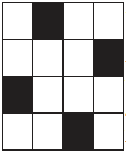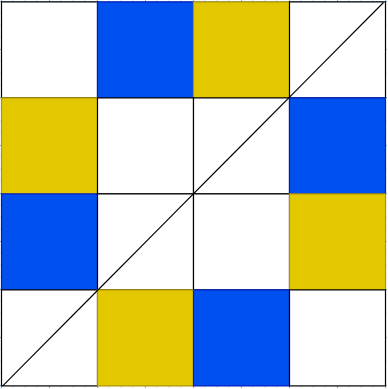# Ex.14.1 Q5 Symmetry - NCERT Maths Class 7

Go back to  'Ex.14.1'

## Question

Copy the figure given here. Take any one diagonal as a line of symmetry and shade a few more squares to make the figure symmetric and diagonal. Is there more than one way to do that? Will the figure be symmetric about both the diagonals?Video Solution
Symmetry
Ex 14.1 | Question 5

## Text Solution

What is known?

A figure with some part shaded.

What is unknown?

To make the given figure symmetric and diagonal.

Is there more than one way to do that?

Will the figure be symmetric about both the diagonals?

Reasoning:

Take any one diagonal as a line of symmetry and shade a few more squares to make the figure symmetric and diagonal.

Steps:Yes, the figure has more than one line of symmetry.

Yes, the figure will be symmetric about both the diagonals.

Learn from the best math teachers and top your exams

• Live one on one classroom and doubt clearing
• Practice worksheets in and after class for conceptual clarity
• Personalized curriculum to keep up with school